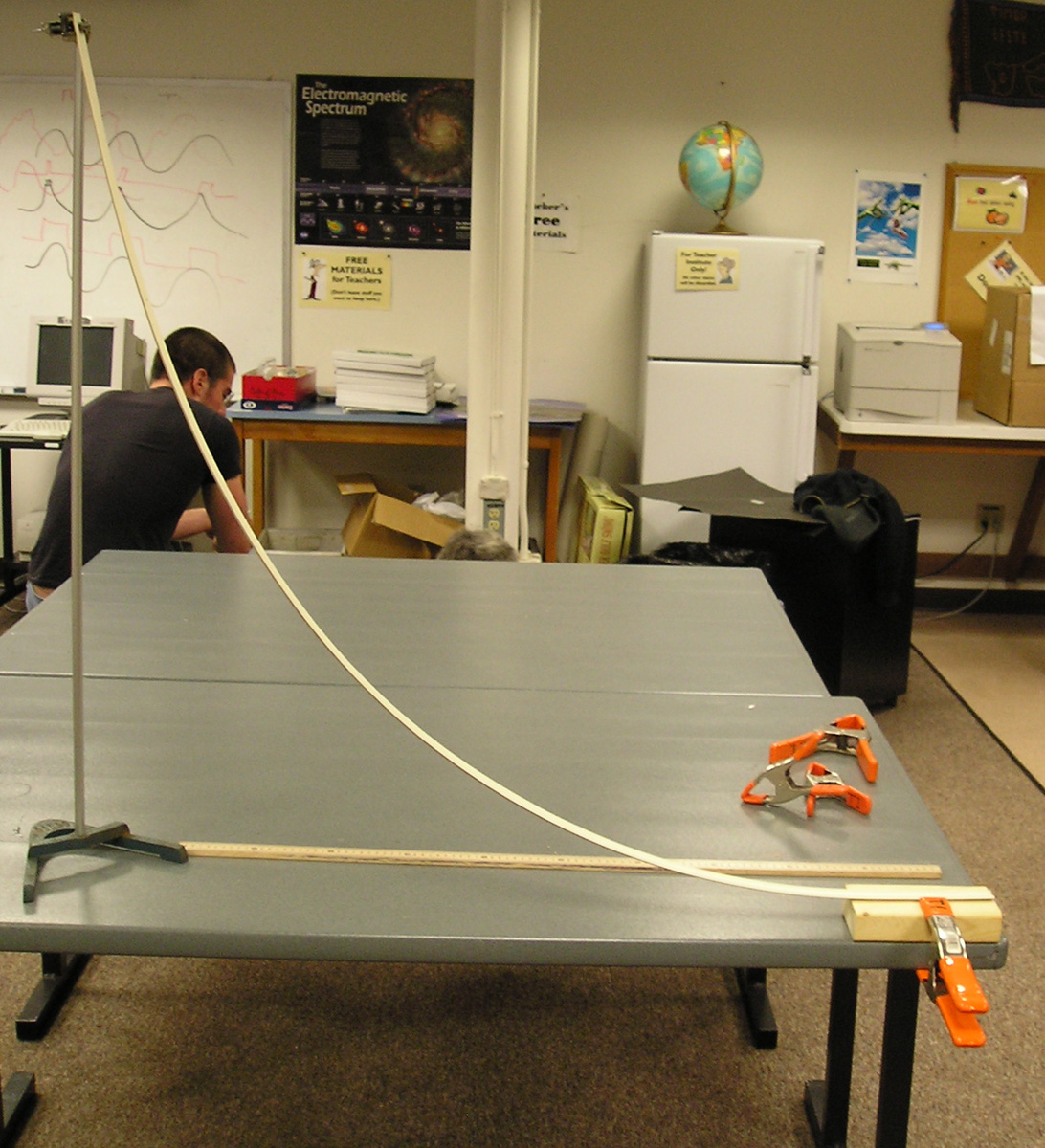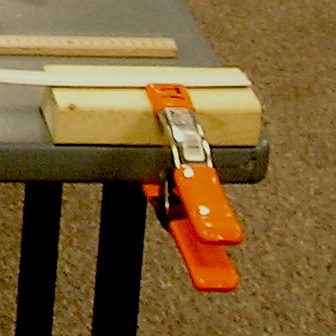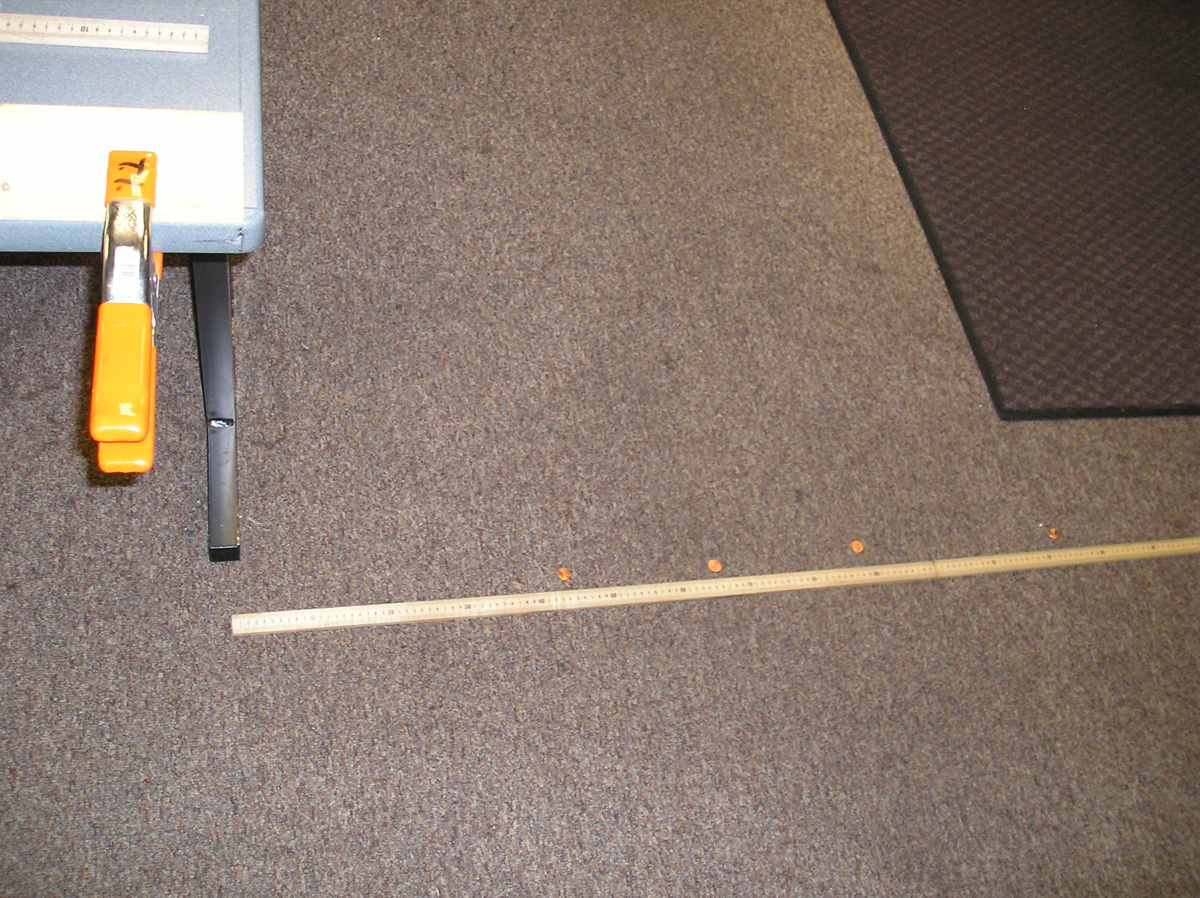Curved Track Roll

The shape mattersThe split PVC ramp

Introduction

A PVC pipe can be sliced in half or in quarters on a bandsaw then shaped into curves to explore the motion of roling balls . Shaping the track can allow the exploration of the conversion of potential to kinetic energy, the operation of tides, and motion along a shape that provides the fastest travel time between two points.

Material

a marble or ball bearing about 1/2 inch (1 cm) in diameter
6 feet ( 2 meters) of Schedule 40 3/4 inch PVC tubing (You can build a longer ramp by using more PVC)
2.5 foot (30 cm) of 1 x 2 wood
20 #10 x 1/2" flat head woodscrews
a bandsaw
a drill with bits to drill a pilot hole in the PC and the wood, and to countersink the head of the woodscrew.
stopwatch
tape measure
or meter stick
for the gravity rainbow exhibit a C-clamp or equivalent to clamp a board to a table.

Assembly

Make the trough

Mark a line along the length of the PVC pipe. Use the bandsaw to cut the PVC in half along this line. (Optional, you can cut the resulting troughs in half again.)

Make the wood blocks

Cut the 2.5 foot long 1x2 wood into 10, three inch long pieces.

Assemble the troughs

For the ball launcher activity attach one block to one end of the trough. Screw through the trough and into the wood, make sure the screw heads are countersunk so the ball can roll over them smoothly. Insert two screws through the PVC into the wide side of each wood mount. Then clamp the wood to a table. Clamp the other end of the PC trough to a ring stand.The trough is screwed to the board which is clamped to the table.

For the brachistochrone and tide explorations attach five blocks to each PVC trough. One at each end, one in the middle, and one each at the 1/4 and 3/4 mark.Screw through the trough and into the wood, make sure the screw heads are countersunk so the ball can roll over them smoothly. Insert two screws through the PVC into the long narrow side of each wood mount. Then screw the wood to a plywood square to create the appropriate shape.

Gravity's Rainbow

To Do and Notice/What's Going On?

Clamp the block at the end of the ramp to the table so that the end of the ramp is level. Use a ringstand or other device to raise the far end of the ramp above the table.

Roll balls down the ramp startng at different heights and watch how they move. Basicaly the potential energy of the ball based on its height above the end of the ramp is converted into kinetic energy as the ball leaves the ramp. The kinetic energy means that the ball leaves the ramp with a horizontal velocity. As soon as the ball leaves the ramp it starts accelerating downward under gravity until it hits the floor. For all initial heights and all launch velocities the ball takes the same time to drop down and hit a level floor. During this time it travels with a constant horizontal velocity. The higher the launch height, the faster the launch velocity and the further the ball travels before it hits the floor.

The potential energy of the ball, U, is mgh where m is the mass of the ball, g is the acceleration of gravity which we will round off to 10 m/s^2 and h is the height of the starting point of the ball bearing minus the height of the ball bearing as it leaves the ramp. If we choose 10 cm to be the height of the ramp at our first start point above the end of the ramp then an interesting thing happens. The potential energy is U = mgh = m*10m/s^2 * 0.1m = m joules.(Where m is measured in kilograms, we will show in the math root below that the mass of the ball cancels out of the problem so any mass ball gives the same answer, so we can either ignore the mass of our ball or choose the mass of our ball. Let's choose 1 kg. This from 10 cm the potential energy will be 1 joule. This will result in a kinetic energy proportional to the velocity of the ball squared, v^2. The velocity of launch will thus be V0. The ball will drop for a time t until it hits the floor, and the distance from the end of the track until it hits the floor will be d0 = V0*t. We launched a ball from a 10 cm hight and the distance it travelled from the end of the ramp was d0 = 40 cm.

Now consider quadrupling the height to 40 cm. Remember that the launch velocity, call it V4, squared is proportional to the initial height. So V4 = square root of 4 * V0 = 2 V0. Thus we predict that the ball will go twice as far since ity falls for the same time and starts out twice as fast. When we did this experiment the ball went 78 cm, almost twice as far.The coins mark the landing points for balls launched from 10 cm, 20 cm,30 cm and 40 cm heights. Notice that the coin launched from 20 cm travelled 1.4 times as far as the coin launched from 10 cm, and remember that the square root of 2 is 1.4

Math Root?

The total kinetic energy of the ball as it leaves the ramp has 2 parts, the kinetic energy of translation, KE = 1/2 mv^2, plus the kinetic energy of rotation, KER = 1/2 I w^2,

Now I is the moment of  inertia of a solid sphere which is I = 0.4 mr^2 where r is the radius of the ball.

And w is the angular velocity of the ball in radians per second. Since the ball is rolling without slipping the linear velocity of the ball,v, is related to the angular velocity, w,in an interesting way,  v = wr. Thus the kinetic energy of rotation is KER = 1/2 I w^2 = 1/2 0.4 mr^2 v^2/r^2, oh frabjous joy! the radius of the ball cancels out as will the mass in the next steps.

So seting the potential energy equal to the total kinetic energy gives

mgh = 1/2 m v^2 + 0.2 m v^2   the m cancels and we can gather terms to make

gh = 0.7 v^2 or solving for v

v = (gh/0.7)^0.5  and indeed launch velocity is proportional to the square root of height.

(Note that if we had an object that slid down frictionlessly all the energy would go into linear kinetic energy and the expression would be v = (gh/0.5)^0.5  the resulting error in the predicted velocity and therefor distance would be about 20% so we muct account for rotational energy, unless we use the technique mentioned in the to do and notice which automatically takes it into account.)

The fall time,tf, depends on the height of the launch ramp above the floor, H. and H = 1/2 g*tf^2 so tf = (2H/g)^0.5

The distance travelled for our first launch at V0 is thus d = V0 * tf = (gh/0.7)^0.5 * (2H/g)^0.5  = (hH/0.7)^0.5 which is interesting because the ball goes the same distance on any planet!

to check our result note d = (0.1 * 0.7/0.7)^0.5 = 0.32 m.

Going Further

 Scientific Explorations with Paul Doherty © 2011 19 Oct 2011